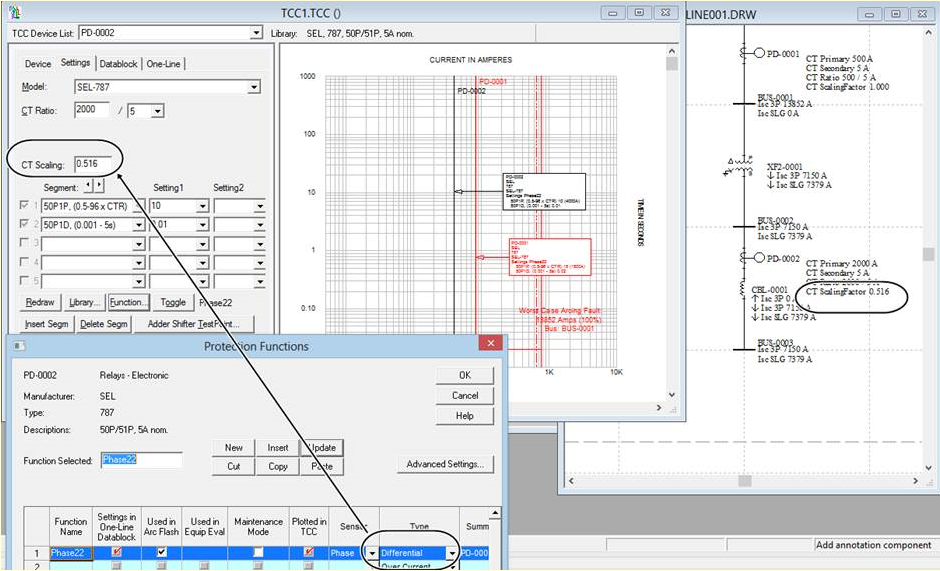# CT Scaling in Relays

The CT scaling factor is only applied to relays set as differential. This field will appear in the component editor or Captor TCC when the function is set to differential. It provides correction factors for the relay CT ratios. For instance, the circuit below has a xfmr sec tap of 1.937 based on the ratio of the 3phase SC (sec/pri) --> 7150A / 13852A = 1 / 1.937 = 0.516. The equivalent CT ratio will be 1032:5. So the relay current in the secondary side is 7150*5/1032 = 1.937*7150*5/2000 = 13852*5/2000.